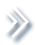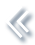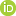####ArchivesReview Articles

## Small area prediction of quantiles for zero-inflated data and an informative sample design

Emily Berg ,

Department of Statistics, Iowa State University, Ames, IA, USAemilyb@iastate.edu

Danhyang Lee

Department of Information Systems, Statistics and Management Science, University of Alabama, Tuscaloosa, AL, USAPages 114-128 | Received 31 Dec. 2018, Accepted 07 Sep. 2019, Published online: 28 Sep. 2019,
• Abstract
• References
• Citations

ABSTRACT

The Conservation Effects Assessment Project (CEAP) is a survey intended to quantify soil and nutrient loss on cropland. Estimates of the quantiles of CEAP response variables are published. Previous work develops a procedure for predicting small area quantiles based on a mixed effects quantile regression model. The conditional density function of the response given covariates and area random effects is approximated with the linearly interpolated generalised Pareto distribution (LIGPD). Empirical Bayes is used for prediction and a parametric bootstrap procedure is developed for mean squared error estimation. In this work, we develop two extensions of the LIGPD-based small area quantile prediction procedure. One extension allows for zero-inflated data. The second extension accounts for an informative sample design. We apply the procedures to predict quantiles of the distribution of percolation (a CEAP response variable) in Kansas counties.

## References

1. Battese, G., Harter, R., & Fuller, W. (1988). An error-components model for prediction of county crop areas using survey and satellite data. Journal of the American Statistical Association83(401), 28–36. doi: 10.1080/01621459.1988.10478561 [Taylor & Francis Online][Web of Science ®], [Google Scholar]
2. Berg, E., & Chandra, H. (2014). Small area prediction for a unit-level lognormal model. Computational Statistics & Data Analysis78, 159–175. doi: 10.1016/j.csda.2014.03.007 [Crossref][Web of Science ®], [Google Scholar]
3. Berg, E., & Lee, D. (2019a). Prediction of small area quantiles for the conservation effects assessment project using a mixed effects quantile regression model. Annals of Applied Statistics, Accepted. [Google Scholar]
4. Berg, E., & Lee, D (2019b). Supplement to “Small Area Prediction of Quantiles for Zero-Inflated Data and an Informative Sample Design.” Supplementary material. [Google Scholar]
5. Breslow, N. E., & Clayton, D. G. (1993). Approximate inference in generalized linear mixed models. Journal of the American Statistical Association88(421), 9–25. [Taylor & Francis Online][Web of Science ®], [Google Scholar]
6. Buchinsky, M., & Hahn, J. (1998). An alternative estimator for the censored quantile regression model. Econometrica66, 653–671. doi: 10.2307/2998578 [Crossref][Web of Science ®], [Google Scholar]
7. Chambers, R., & Tzavidis, N. (2006). M-quantile models for small area estimation. Biometrika93(2), 255–268. doi: 10.1093/biomet/93.2.255 [Crossref][Web of Science ®], [Google Scholar]
8. Chandra, H., & Sud, U. C. (2012). Small area estimation for zero-inflated data. Communications in Statistics-Simulation and Computation41(5), 632–643. doi: 10.1080/03610918.2011.598991 [Taylor & Francis Online][Web of Science ®], [Google Scholar]
9. Chernozhukov, V., Fernandez-Val, I., & Galichon, A. (2009). Improving point and interval estimators of monotone functions by rearrangement. Biometrika96, 559–575. doi: 10.1093/biomet/asp030 [Crossref][Web of Science ®], [Google Scholar]
10. Dreassi, E., Petrucci, A., & Rocco, E. (2014). Small area estimation for semicontinuous skewed spatial data: An application to the grape wine production in tuscany. Biometrical Journal56(1), 141–156. doi: 10.1002/bimj.201200271 [Crossref][Web of Science ®], [Google Scholar]
11. Hall, P., & Maiti, T. (2006). Nonparametric estimation of mean-squared prediction error in nested-error regression models. The Annals of Statistics34, 1733–1750. doi: 10.1214/009053606000000579 [Crossref][Web of Science ®], [Google Scholar]
12. Jang, W., & Wang, J. (2015). A semiparameteric Bayesian approach for joint-quantile regression with clustered data. Computational Statistics and Data Analysis84, 99–115. doi: 10.1016/j.csda.2014.11.008 [Crossref][Web of Science ®], [Google Scholar]
13. Kim, J. K., & Yu, C. L. (2011). A semiparametric estimation of mean functionals with nonignorable missing data. Journal of the American Statistical Association106(493), 157–165. doi: 10.1198/jasa.2011.tm10104 [Taylor & Francis Online][Web of Science ®], [Google Scholar]
14. Koenker, R. (2005). Quantile regression. New York: Cambridge University Press. doi: 10.1017/CBO9780511754098 [Crossref], [Google Scholar]
15. Koenker, R., & Ng, P. (2005). Inequality constrained quantile regression. Sankhya: The Indian Journal of Statistics67, 418–440. [Google Scholar]
16. Lahiri, S. N., Maiti, T., Katzoff, M., & Parsons, V. (2007). Resampling-based empirical prediction: An application to small area estimation. Biometrika94, 469–485. doi: 10.1093/biomet/asm035 [Crossref][Web of Science ®], [Google Scholar]
17. Lyu, X (2018). Empirical Bayes small area prediction of sheet and rill erosion under a zero-inflated lognormal model (Master's Thesis). Iowa State University. [Google Scholar]
18. Opsomer, J. D., Claeskens, G., Ranalli, M. G., Kauermann, G., & Breidt, F. J. (2008). Non-parametric small area estimation using penalized spline regression. Journal of the Royal Statistical Society: Series B (Statistical Methodology)70(1), 265–286. doi: 10.1111/j.1467-9868.2007.00635.x [Crossref], [Google Scholar]
19. Pfeffermann, D., & Sverchkov, M. (2007). Small-area estimation under informative probability sampling of areas and within the selected areas. Journal of the American Statistical Association102(480), 1427–1439. doi: 10.1198/016214507000001094 [Taylor & Francis Online][Web of Science ®], [Google Scholar]
20. Pfeffermann, D., Terryn, B., & Moura, F. A. (2008). Small area estimation under a two-part random effects model with application to estimation of literacy in developing countries. Survey Methodology34(2), 235–249. [Web of Science ®], [Google Scholar]
21. Powell, J. L. (1986). Censored regression quantiles. Journal of Econometrics32(1), 143–155. doi: 10.1016/0304-4076(86)90016-3 [Crossref][Web of Science ®], [Google Scholar]
22. Rao, J. N., & Molina, I. (2015). Small area estimation. Hoboken, NJ: John Wiley & Sons. [Crossref], [Google Scholar]
23. Searle, S. R., Casella, G., & McCulloch, C. E. (1992). Variance components. New York: John Wiley & Sons. [Crossref], [Google Scholar]
24. Sinha, S. K., & Rao, J. N. K. (2009). Robust small area estimation. Canadian Journal of Statistics37(3), 381–399. doi: 10.1002/cjs.10029 [Crossref][Web of Science ®], [Google Scholar]
25. Smith, A. F., & Gelfand, A. E. (1992). Bayesian statistics without tears: A sampling-resampling perspective. The American Statistician46(2), 84–88. [Taylor & Francis Online][Web of Science ®], [Google Scholar]
26. Verret, F., Rao, J. N. K., & Hidiroglou, M. A. (2015). Model-based small area estimation under informative sampling. Survey Methodology41(2), 333–347. [Web of Science ®], [Google Scholar]
27. Wang, J., Fuller, W. A., & Qu, Y. (2008). Small area estimation under a restriction. Survey Methodology34, 29–36. [Web of Science ®], [Google Scholar]
28. Wischmeier, W. H., & Smith, D. D (1978). Predicting rainfall erosion losses a guide to conservation planning. U.S. Department of Agriculture, Agriculture Handbook No. 537. [Google Scholar]
29. You, Y., & Rao, J. N. K. (2002). A pseudo empirical best linear unbiased prediction approach to small area estimation using survey weights. Canadian Journal of Statistics30(3), 431–439. doi: 10.2307/3316146 [Crossref][Web of Science ®], [Google Scholar]